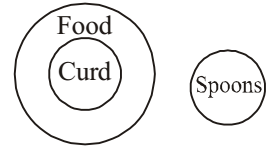## Logical Venn Diagram

#### Logical Venn Diagram

1. Indicate which figure will best represent the relationship amongst the three:
Legumes Seeds, Peas, Kidney Beans

1. As per the given above question , we can say that
Pea is different from kidney bean. But both are Leguminous seeds.

##### Correct Option: B

As per the given above question , we can say that
Pea is different from kidney bean. But both are Leguminous seeds.
From above it is clear that Peas and Kidney Beans belong to Legumes Seeds .Hence , required answer is option ( B ) .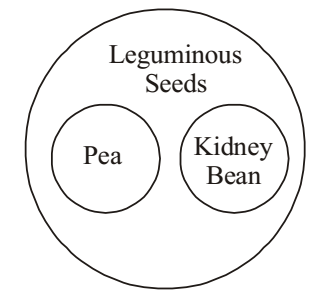1. In the given figure, circles represent students studying 3 different subjects. How many students study all the 3 subjects?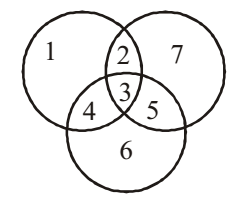1. From above given diagram , we can say that
The number ‘3’ is common to all the three circles.

##### Correct Option: B

From above given diagram , we can say that
The number ‘3’ is common to all the three circles.
Hence , 3 students study all the three subjects .

1. The diagram represents Teachers, Singers and Players. Study the diagram and find out how many teachers are also singers.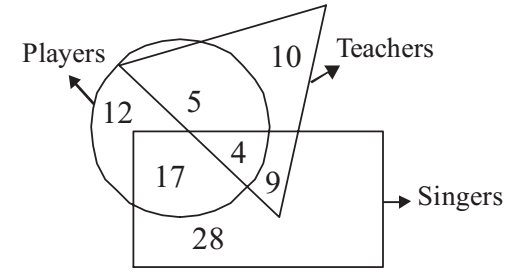1. From the given diagram , we can see that
Number of teachers who are also singers = 9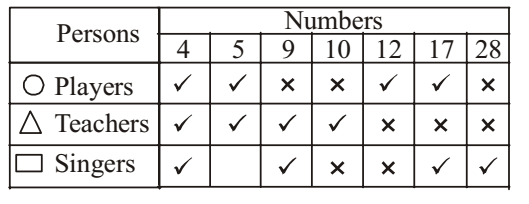##### Correct Option: C

From the given diagram , we can see that
Number of teachers who are also singers = 9
Hence , 9 teachers are also singers.1. In a group of persons, 11 persons speak Kannada, 20 persons speak Tamil and 11 persons speak Telugu. In that group, if two persons speak 2 languages and 1 person speak all the languages, then how many persons are there in the group?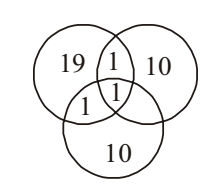1. According to question ,
Let us assume Number of Kannada = K , Number of Tamil = T and Number of Telugu = Te
Here , n(K) = 11; n(T) = 20; n(Te) = 11
n(K ∩ T ∩ Te) = 1
n(K ∩ T) = n(T ∩ Te) = n(Te ∩ K) = 2
n(K U T U Te) = n(T) + n(K) + n(Te) - n(K ∩ T) - n(T ∩ Te) - n(Te ∩ K) + n(K ∩ T ∩ Te)

##### Correct Option: C

According to question ,
Let us assume Number of Kannada = K , Number of Tamil = T and Number of Telugu = Te
Here , n(K) = 11; n(T) = 20; n(Te) = 11
n(K ∩ T ∩ Te) = 1
n(K ∩ T) = n(T ∩ Te) = n(Te ∩ K) = 2
n(K U T U Te) = n(T) + n(K) + n(Te) - n(K ∩ T) - n(T ∩ Te) - n(Te ∩ K) + n(K ∩ T ∩ Te)
n(K U T U Te) = 20 + 11 + 11 - 2 - 2 - 2 + 1 = 37

1. Identify the diagram that best represents the relationship among classes given below :
Food, Curd, Spoons

1. According to question , we can say that
Food and Curd belong to same class , but Spoons belong to other class .

##### Correct Option: D

According to question , we can say that
Food and Curd belong to same class , but Spoons belong to other class .
From above it is clear that required answer will be option D .So , option D is correct .